# Solid geometry, stereometry

Solid geometry is the name for the geometry of three-dimensional Euclidean space.

Stereometry involves the measurements of volumes of various solid figures (three-dimensional figures), including pyramids, prisms, and other polyhedrons; cylinders; cones; truncated cones; and balls bounded by spheres.

#### Number of problems found: 1102

• An experiment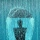The three friends agreed to the experiment. At the same time, they all took out an empty cylindrical container on the windowsill and placed it so that it was horizontal. Everyone lives in a different village, and each used a container with a different bot
• Waste container eco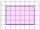The waste box has the shape of a block with dimensions of 1.8 m, 1.5 m, 1.2 m. 0.25 m3 of waste is added to it every day. How many days will it be filled?
• Right-angled triangle baseFind the volume and surface area of a triangular prism with a right-angled triangle base if the length of the prism base legs are 7.2 cm and 4.7 cm and the height of a prism is 24 cm.
• How toHow to find a total surface of a rectangular pyramid if each face is to be 8 dm high and the base is 10 dm by 6 dm.
• Empty aquariumHow much does an empty aquarium weigh with dimensions: length = 40 cm, width = 30 cm, height = 20 cm, if 1 dm2 of glass weighs 300 g? Calculate its weight in kilograms.
• Cylinder container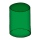If the cylinder-shaped container is filled with water to a height of 5 dm, it contains 62.8 hectoliters of water. Calculate the diameter of the bottom of the container. Use the value π = 3.14.
• Frustrum - volume, areaCalculate the surface and volume of the truncated cone, the radius of the smaller figure is 4 cm, the height of the cone is 4 cm and the side of the truncated cone is 5 cm.
• Mr. Gardener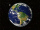Mr. Gardener wants to make wood for the balcony. Boxes. Each will have the shape of a perpendicular prism with a square base, the height is limited to 60 cm. Each container will be filled with soil by pouring the whole bag of substrate sold in a package w
• Frustrum - volume, area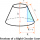Calculate the surface and volume of a truncated rotating cone with base radii of 8 cm and 4 cm, height 5 cm.
• Tower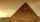Charles built a tower of cubes with an edge 2 cm long. In the lowest layer there were 6 cubes (in one row) in six rows, in each subsequent layer always 1 cube and one row less. What volume in cm3 did the whole tower have?
• Regular square prismThe volume of a regular square prism is 192 cm3. The size of its base edge and the body height is 1: 3. Calculate the surface of the prism.
• An architect 2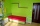An architect is designing a house. He wants the bedroom to have the dimensions of 8 ft by 4 ft by 7 ft. The architect doubles all three dimensions to create the den. Does that mean the den will have double the volume of the bedroom? First, find the volume
• The volleyball ball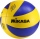The volleyball ball can have a circumference after inflation of at least 650 max 750 mm. What volume of air can this ball hold, if its circumference is the average of the minimum and maximum inflation of the ball.
• The solarThe solar heating tank has the shape of a rotating cylinder. When the tank is in a horizontal position, the water in the tank wets 4/5 each tank bases. If we place the tank in a vertical position, the water in the tank will reach up to 1.2 m. Calculate th
• A box 2A box has a length of 4 1/2 inches, a width of 3 2/3 inches and a height of 8 1/4 inches. What is the volume of the box?
• Surveyors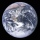Surveyors mark 4 points on the surface of the globe so that their distances are the same. What is their distance from each other?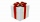How much wrapping paper is needed to wrap a cube-shaped gift with edge measuring 15 cm?
• Trapezoidal base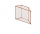Calculate the surface and volume of a quadrilateral prism with a trapezoidal base, where a = 7 cm, b = 4 cm, c = 5 cm, d = 4 cm, height of trapezium v = 3.7 cm and the height of the prism h = 5 cm.
• Axial sectionCalculate the volume and surface of a cone whose axial section is an equilateral triangle with side length a = 18cm.
• Top-open tank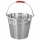The top-open tank has the shape of a truncated rotating cone, which stands on a smaller base. The tank's volume is 465 m3, the radii of the bases are 4 m and 3 m. Find the depth of the tank.

Do you have an exciting math question or word problem that you can't solve? Ask a question or post a math problem, and we can try to solve it.

We will send a solution to your e-mail address. Solved examples are also published here. Please enter the e-mail correctly and check whether you don't have a full mailbox.

Please do not submit problems from current active competitions such as Mathematical Olympiad, correspondence seminars etc...